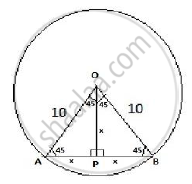# A chord of a circle of radius 10 em subtends a right angle at its centre. The length of the chord (in em) is - Mathematics

A chord of a circle of radius 10 em subtends a right angle at its centre. The length of the chord (in em) is

(A) 5sqrt 2

(B) 10 sqrt2

(C)5/sqrt2

(D) 10sqrt 3

#### SolutionGiven ∠ AOB is given as 90º
ΔAOB is an isosceles triangle since OA = OB
Therefore ∠OAB = ∠OBA = 45º
Thus ∠ AOP = 45º and ∠ BOP = 45º
Hence ΔAOP and ΔBOP also are isosceles triangles

AP = OP and OP PB
In ΔAOP

x2 +  x2 =  102 [Pythagoras theorem]
Thus 2 x2 = 100
x=5√2
Hence length of chord AB = 2 x = 5√2+5√2=10√2

Concept: Concept of Circle - Centre, Radius, Diameter, Arc, Sector, Chord, Segment, Semicircle, Circumference, Interior and Exterior, Concentric Circles
Is there an error in this question or solution?
2013-2014 (March) All India Set 2

Share